[Home]

#### A Sixth GeneralizationWe continue in the following page with a binomial summation formula of the more general linear second order recurrence equation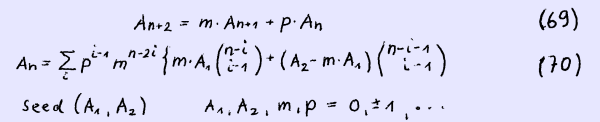We proof by induction writing An in full yields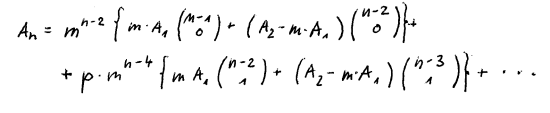Obviously A1 = m-1 · m · A1 is correct.

We assume An to be correct for n = r and n = r + 1 that is Ar and Ar+1.

Then we state Ar+2 = m Ar+1 + p Ar to be correct.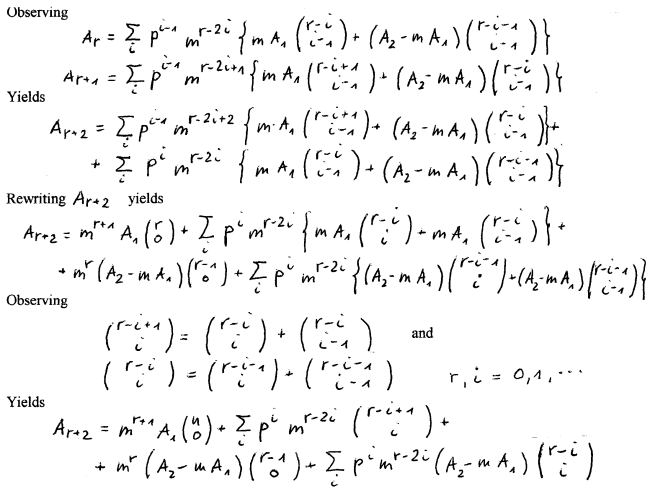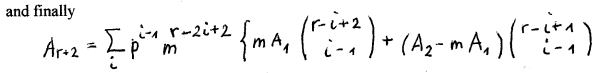This is just our statement when replacing n by r + 2. So our binomial summation formula holds for any r.

From (70) some other formulae emerge. A linear second order recurrence formula in conjunction with a binomial summation formula for a serie composed of every k-th element of the generalized Fibonacci serie Gkn+e is given below.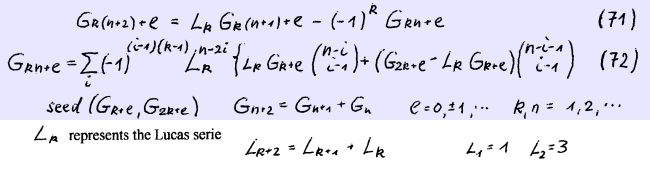We proof the recurrence formula by using Binets formula for Gk(n+1)+e and Lk obtaining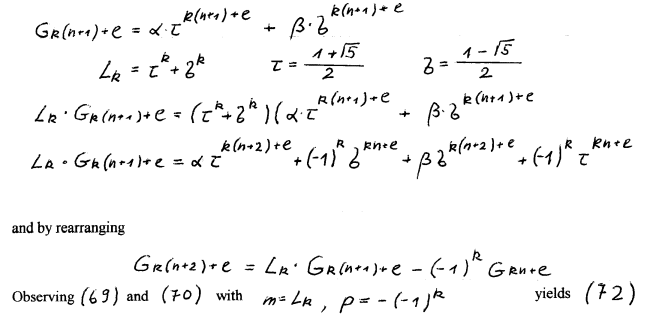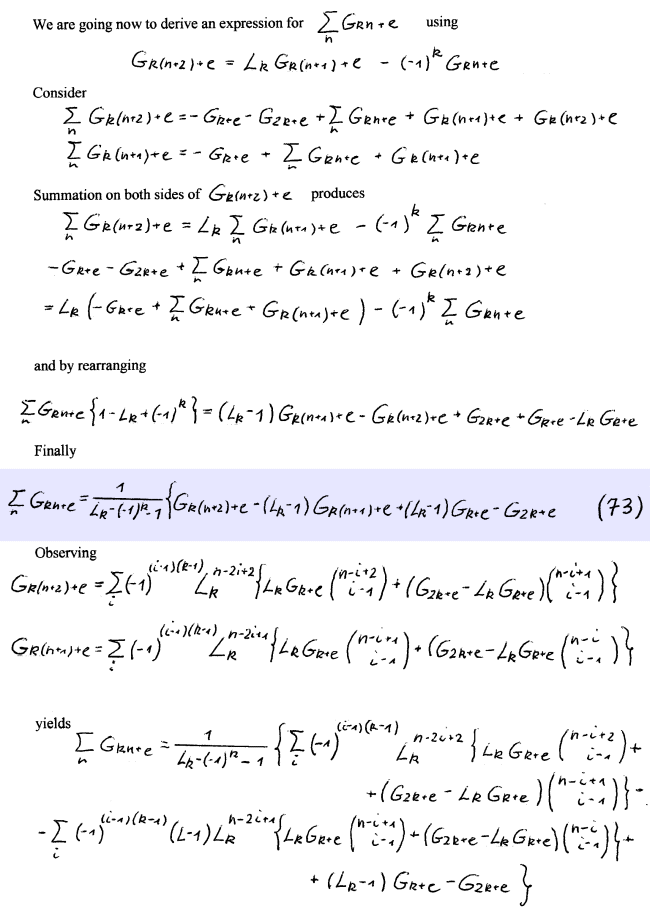And by rearranging the above binomial summation formula we obtain finally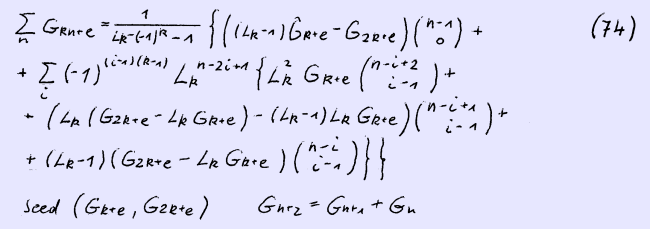We consider now the more general case Akn+e. Both the recurrence formula and the corresponding binomial summation formula are given below.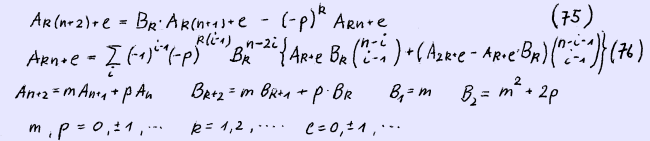We proof first (75) in a very similar way then (71)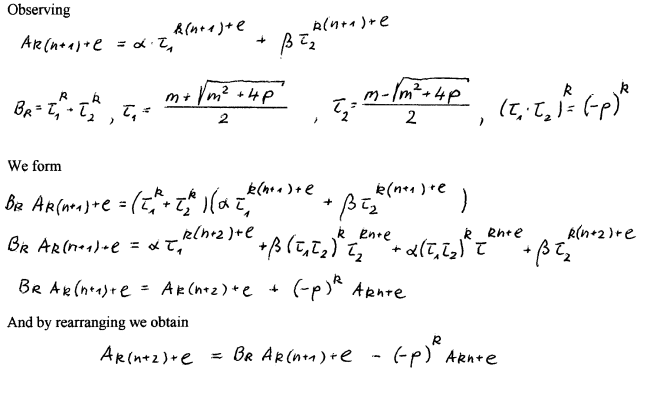Observing (69) and (70) yields (76).

In particular when m = p = 1 we obtain formulae (71) and (72).

In our next page we are concerned with linear third order recurrence formulae, A recurrence formula

An+3 = An+2 + An+1 + An

has often been called a Tribonacci sequence. We are concerned there with a sequence composed of the squares of Fn that is F2n which leads to another linear third order recurrence formula. You are kindly invited to this page.